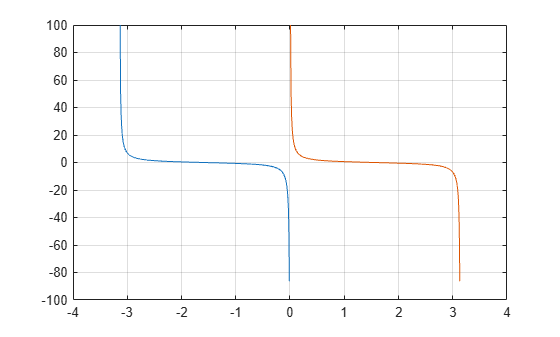# cot

## Syntax

``Y = cot(X)``

## Description

example

````Y = cot(X)` returns the cotangent of elements of `X`. The `cot` function operates element-wise on arrays. The function accepts both real and complex inputs. For real values of `X`, `cot(X)` returns real values in the interval [-∞, ∞].For complex values of `X`, `cot(X)` returns complex values. ```

## Examples

collapse all

Plot the cotangent function over the domain $-\pi and $0 .

```x1 = -pi+0.01:0.01:-0.01; x2 = 0.01:0.01:pi-0.01; plot(x1,cot(x1),x2,cot(x2)), grid on```Calculate the cotangent of the complex angles in vector `x`.

```x = [-i pi+i*pi/2 -1+i*4]; y = cot(x)```
```y = 1×3 complex 0.0000 + 1.3130i -0.0000 - 1.0903i -0.0006 - 0.9997i ```

## Input Arguments

collapse all

Input angle in radians, specified as a scalar, vector, matrix, multidimensional array, table, or timetable.

Data Types: `single` | `double` | `table` | `timetable`
Complex Number Support: Yes

## Output Arguments

collapse all

Cotangent of input angle, returned as a real-valued or complex-valued scalar, vector, matrix, multidimensional array, table, or timetable.

collapse all

### Cotangent Function

The cotangent of an angle, α, defined with reference to a right angled triangle is

.The cotangent of a complex argument α is

`$\text{cot}\left(\alpha \right)=\frac{i\left({e}^{i\alpha }+{e}^{-i\alpha }\right)}{\left({e}^{i\alpha }-{e}^{-i\alpha }\right)}\text{\hspace{0.17em}}.$`

.

## Tips

• In floating-point arithmetic, `cot` is a bounded function. That is, `cot` does not return values of `Inf` or `-Inf` at points of divergence that are multiples of `pi`, but a large magnitude number instead. This stems from the inaccuracy of the floating-point representation of π.

## Version History

Introduced before R2006a

expand all